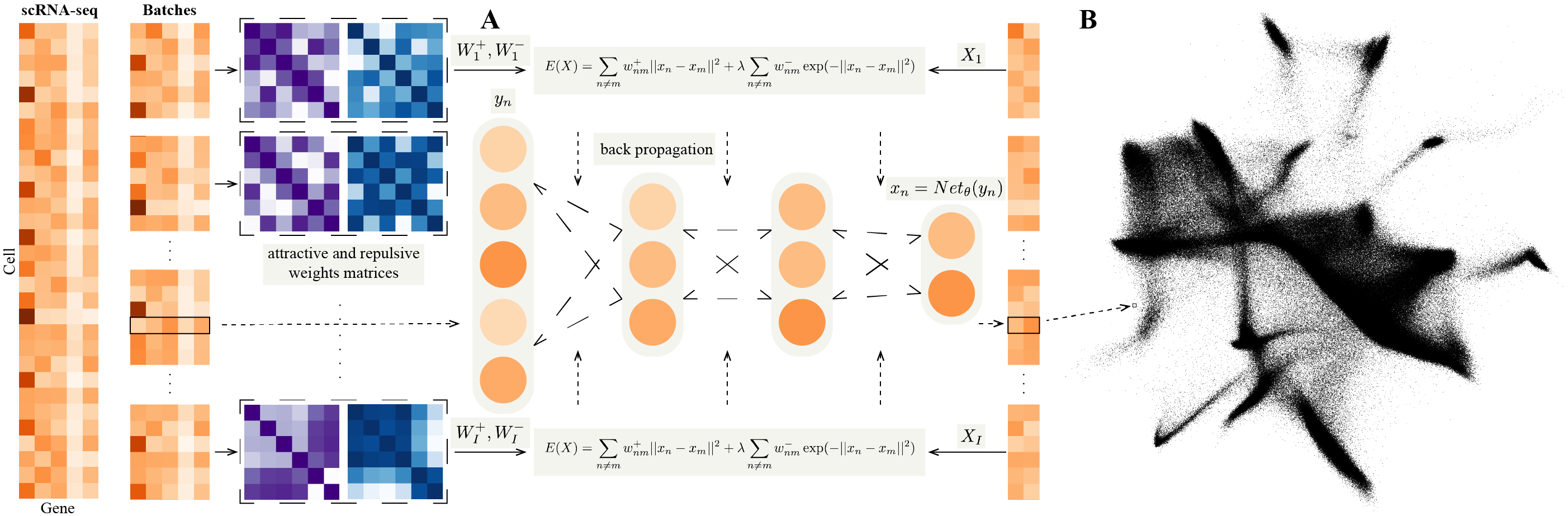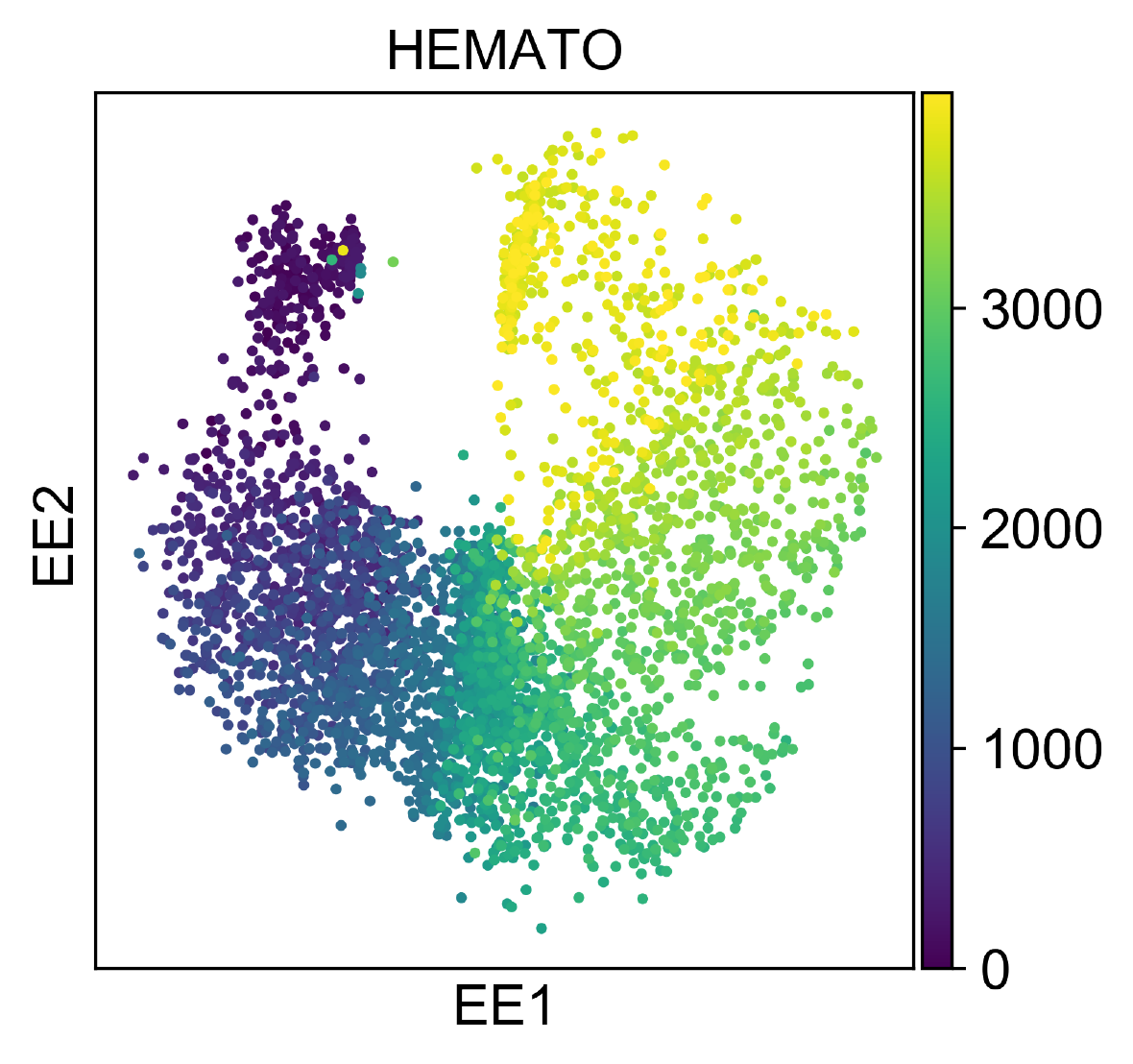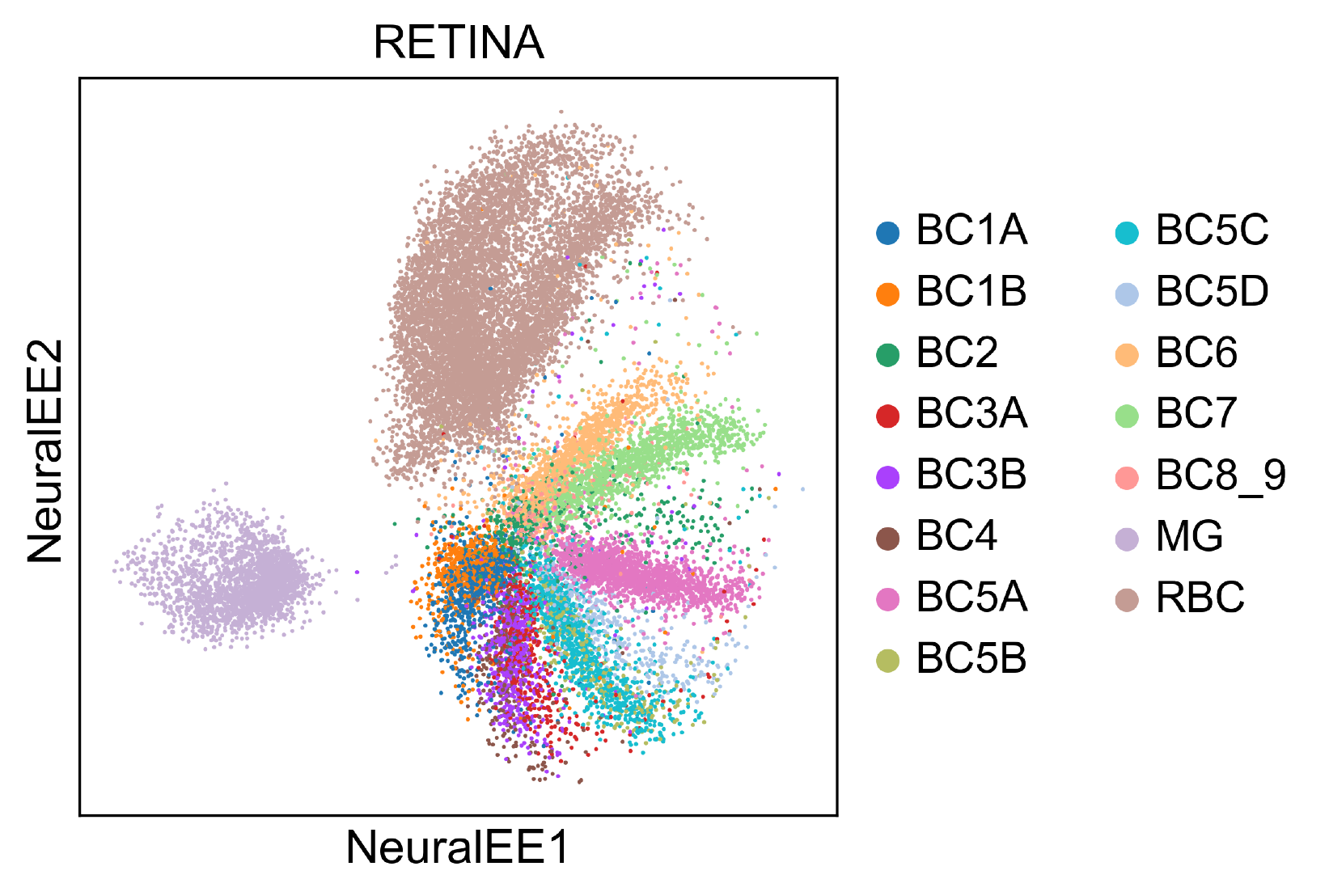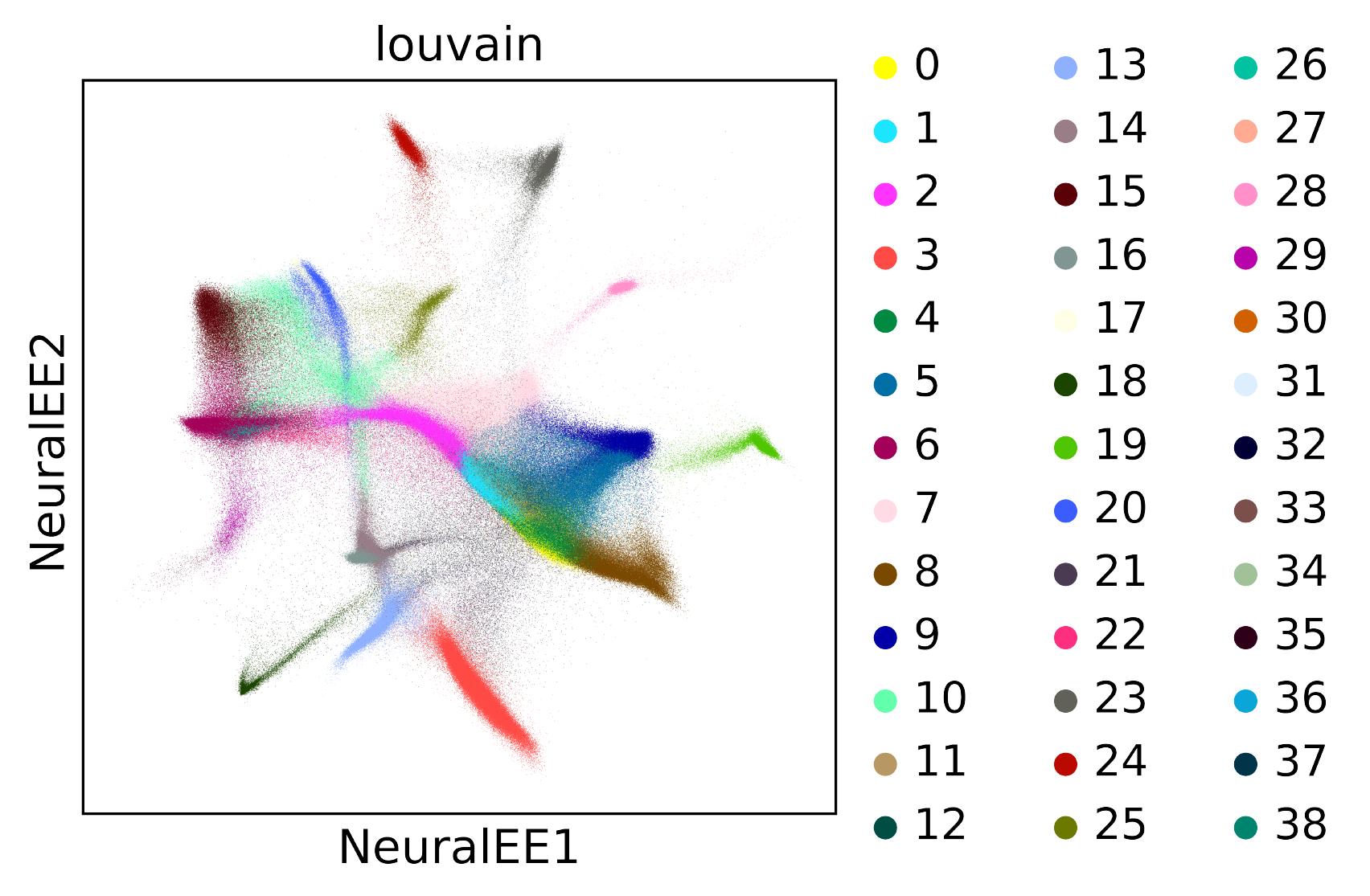# Welcome to NeuralEE’s documentation!¶

## NeuralEE¶This is an applicable version for NeuralEE.

1. The datasets loading and preprocessing module is modified from scVI v0.3.0.
2. Define NeuralEE class and some auxiliary function, mainly for cuda computation, except like entropic affinity calculation which is quite faster computed on cpu.
3. General elastic embedding algorithm on cuda is given based on matlab code from Max Vladymyrov.
4. Add some demos of notebook helping to reproduce.

### Installation¶

1. Install Python 3.7.
2. Install PyTorch. If you have an NVIDIA GPU, be sure to install a version of PyTorch that supports it. NeuralEE runs much faster with a discrete GPU.
3. Install NeuralEE through pip or from GitHub:
pip install neuralee

git clone git://github.com/HiBearME/NeuralEE.git
cd NeuralEE
python setup.py install --user


### How to use NeuralEE¶

Our datasets loading and preprocessing module is based on scVI v0.3.0. How to download online datasets or load generic datasets is the same as scVI v0.3.0.

For example, load the online dataset CORTEX Dataset, which consists of 3,005 mouse cortex cells profiled with the Smart-seq2 protocol. To facilitate comparison with other methods, we use a filtered set of 558 highly variable genes as the original paper.

from neuralee.dataset import CortexDataset
dataset = CortexDataset(save_path='../data/')
dataset.log_shift()
dataset.subsample_genes(558)
dataset.standardscale()


Load the h5ad file for BRAIN-LARGE Dataset, which consists of 1.3 million mouse brain cells and has been already preprocessed and remained by 50 principal components.

from neuralee.dataset import GeneExpressionDataset
import anndata


For other generic datasets, it’s also convenient to use GeneExpressionDataset to load them.

1. Embedding

There are a number of parameters that can be set for the NeuralEE class; the major ones are as follows:

• d: This determines the dimension of embedding space, with 2 being default.
• lam: This determines the trade-off parameter of EE objective function. Larger values make embedded points more distributed. In general this parameter should be non-negative, with 1.0 being default.
• perplexity: This determines the perplexity parameter for calculation of the attractive matrix. This parameter plays the same role as t-SNE, with 30.0 being default.
• N_small: This determines the batch-size for the stochastic optimization. Smaller value makes more accurate approximation to the original EE objective function, but needs larger computer memory to save the attractive and repulsive matrices and longer time for optimization. It could be inputted as integer or percentage, with 1.0 being default, which means not applied with stochastic optimization. we recommend to use stochastic optimization when only necessary, such as on BRAIN-LARGE Dataset, which is hard to save the original attractive and repulsive matrices for a normal computer, and if not with stochastic optimization, it could run out of memory.
• maxit: This determines the maximum iteration of optimization. Larger values makes embedded points stabler and more convergent, but consumes longer time, with 500 being default.
• pin_memory: This determines whether to transfer all the matrix to the GPU at once if a GPU is available, with True being default. If it’s True, it could save lots of time of transferring data from computer memory to GPU memory in each iteration, but if your GPU memory is limited, it must be set as False, for each iteration, the matrices of the current iteration are re-transferred to the GPU at the beginning and freed at the end.

The embedding steps are as follows:

a). Calculate attractive and repulsive matrices for the dataset.

If EE and NeuralEE without stochastic optimization will be used, it could be calculated as:

dataset.affinity(perplexity=30.0)


Or NeuralEE with stochastic optimization will be used, for example, 10% samples for each batch, it could be calculated as:

dataset.affinity_split(perplexity=30.0, N_small=0.1, verbose=True)
# verbose=True determines whether to show the progress of calculation.


b). Initialize NeuralEE class.

import torch
# detect whether to use GPU.
device = torch.device('cuda:0' if torch.cuda.is_available() else 'cpu')
NEE = NeuralEE(dataset, d=2, lam=1, device=device)


c). Embedding.

If EE will be used, it could be calculated as:

results_EE = NEE.EE(maxit=50)


If NueralEE will be used, it could be calculated as:

results_NeuralEE = NEE.fine_tune(maxit=50, verbose=True, pin_memory=False)


For reproduction of original paper’s results, check at Jupyter notebooks files.

### Computer memory consuming¶

Computer memory is mainly allocated for saving attractive and repulsive matrices, which is approximately calculated as follows:

$\frac{DataSize \times BatchSize \times 4 \times 2}{1024^3}(GBytes)$

Hyper-parameters selection of NeuralEE on large-scale data is limited on computers with limited memory.

### Examples¶

1. HEMATO

HEMATO Dataset includes 4,016 cells, and provides a snapshot of hematopoietic progenitor cells differentiating into various lineages.

This dataset is quite small, so we directly apply NeuralEE.EE and with (lam =10, perplexity =30). And it could finish in several minutes on CPU, and in several seconds on GPU.1. RETINA

RETINA Dataset includes 27,499 mouse retinal bipolar neurons. Cluster annotation is from 15 cell-types from the original paper.

Size of this dataset is moderate, and EE on CPU could finish in several hours. However, NeuralEE on a normal GPU, equipped with 11G memory, without stochastic optimization could finish in almost 3 minutes. And on a GPU of limited memory, NeuralEE with (N_small =0.5, pin_memory = True) could finish in almost 2 minutes. The follow embedding shows the result of NeuralEE with (lam =10, perplexity =30, N_small =0.5, pin_memory = True).To reproduce this, check at Jupyter notebook for RETINA dataset.

1. BRAIN-LARGE

BRAIN-LARGE Dataset consists of 1.3 million mouse brain cells, and it’s clustered by Louvain algorithm.

This dataset is quite large, so it’s very difficult to apply EE. Instead, we apply NeuralEE with (lam =1, perplexity =30, N_small =5000, maxit =50, pin_memory = False) on a normal GPU, equipped with 11G memory (when set pin_memory as False, It also works on a GPU of limited memory and only uses less than 1G memory). It needs at least 64G computer memory to save data, and it could finish less than half hour.To reproduce this, check at Jupyter notebook for BRAIN-LARGE dataset.

## neuralee.dataset package¶

This module is modified from scVI.

class neuralee.dataset.CortexDataset(save_path='data/', genes_to_keep=[], genes_fish=[], additional_genes=None)[source]

Bases: neuralee.dataset.dataset.GeneExpressionDataset

The Mouse Cortex Cells dataset contains 3005 mouse cortex cells and gold-standard labels for seven distinct cell types. Each cell type corresponds to a cluster to recover. We retain top 558 genes ordered by variance.

Parameters: save_path – Save path of raw data file.

Examples:

gene_dataset = CortexDataset()

preprocess()[source]
static reorder_genes(x, genes, first_genes)[source]

In case the order of the genes needs to be changed: puts the gene present in ordered_genes first, conserving the same order.

class neuralee.dataset.BrainLargeDataset(subsample_size=None, save_path='data/', nb_genes_kept=720, max_cells=None)[source]

Bases: neuralee.dataset.dataset.GeneExpressionDataset

This dataset contains 1.3 million brain cells from 10x Genomics. We randomly shuffle the data to get a 1M subset of cells and order genes by variance to retain first 10,000 and then 720 sampled variable genes.

Parameters: save_path – Save path of raw data file.

Examples:

gene_dataset = BrainLargeDataset()

preprocess()[source]
class neuralee.dataset.RetinaDataset(save_path='data/')[source]

Bases: neuralee.dataset.loom.LoomDataset

The dataset of bipolar cells contains after their original pipeline for filtering 27,499 cells and 13,166 genes coming from two batches. We use the cluster annotation from 15 cell-types from the author.

Parameters: save_path – Save path of raw data file.

Examples:

gene_dataset = RetinaDataset()

class neuralee.dataset.GeneExpressionDataset(Y, batch_indices=None, labels=None, gene_names=None, cell_types=None)[source]

Bases: object

Gene Expression dataset.

Parameters: Y (numpy.ndarray or numpy.matrix) – gene expression matrix. batch_indices – batch indices. if None, set as np.zeros. labels – labels. if None, set as np.zeros. gene_name – gene names. cell_types – cell types.
affinity(aff='ea', perplexity=30.0, neighbors=None)[source]

Affinity calculation.

Parameters: aff ({'ea', 'x2p'}) – affinity used to calculate attractive weights. perplexity – perplexity defined in elastic embedding function. neighbors (int) – the number of nearest neighbors
affinity_split(N_small=None, aff='ea', perplexity=30.0, verbose=False, neighbors=None)[source]

Affinity calculation on each batch.

Preparation for NeuralEE with mini-batch trick.

Parameters: N_small (int or percentage) – size of each batch. aff ({'ea', 'x2p'}) – affinity used to calculate attractive weights. perplexity – perplexity defined in elastic embedding function. verbose (bool.) – whether to show the progress of affinity calculation. neighbors (int) – the number of nearest neighbors
static concat_datasets(*gene_datasets, on='gene_names', shared_labels=True, shared_batches=False)[source]

Combines multiple unlabelled gene_datasets based on the intersection of gene names intersection. Datasets should all have gene_dataset.n_labels=0. Batch indices are generated in the same order as datasets are given. :param gene_datasets: a sequence of gene_datasets object :return: a GeneExpressionDataset instance of the concatenated datasets

download()[source]

download_and_preprocess()[source]

filter_cell_types(cell_types)[source]

update data by given cell types.

Parameters: cell_types (numpy.ndarray) – indices(np.int) or cell-types names(np.str).
filter_genes(gene_names_ref, on='gene_names')[source]

update dataset by given subset of genes’ names.

Parameters: gene_names_ref – subset of genes’ names.
static get_attributes_from_list(Xs, list_batches=None, list_labels=None)[source]

acquire dataset from lists.

static get_attributes_from_matrix(X, batch_indices=0, labels=None)[source]

acquire dataset from matrix.

log_shift()[source]

lambda: x -> log(1+x)

map_cell_types(cell_types_dict)[source]

A map for the cell types to keep, and optionally merge together under a new name (value in the dict).

Parameters: cell_types_dict – a dictionary with tuples (str or int) as input and value (str or int) as output
merge_cell_types(cell_types, new_cell_type_name)[source]

Merge some cell types into a new one, a change the labels accordingly.

Parameters: cell_types (numpy.ndarray) – indices(np.int) or cell-types names(np.str). new_cell_type_name (numpy.ndarray) – indices(np.int) or cell-types names(np.str).
remove_zero_sample()[source]

remove zero expression samples.

standardscale()[source]

standard scaling across gene.

subsample_cells(size=1.0)[source]

update dataset by filtering cells according to variance.

Parameters: size (int or percentage) – subsample size.
subsample_genes(new_n_genes=None, subset_genes=None)[source]

update dataset by filtering genes according to variance.

Parameters: new_n_genes – number of genes remain. if subset_genes not provided. subset_genes – subset of cells’indexes.
update_cells(subset_cells)[source]

update dataset by given subset of cells’ indexes.

Parameters: subset_cells – subset of cells’indexes.
update_genes(subset_genes)[source]

update dataset by given subset of genes’ indexes.

Parameters: subset_genes – subset of genes’ indexes.
class neuralee.dataset.CiteSeqDataset(name='cbmc', save_path='data/citeSeq/')[source]

Bases: neuralee.dataset.dataset.GeneExpressionDataset

preprocess()[source]
class neuralee.dataset.BrainSmallDataset(save_path='data/')[source]

Bases: neuralee.dataset.dataset10X.Dataset10X

This dataset consists in 9,128 mouse brain cells profiled using 10x Genomics is used as a complement of PBMC for our study of zero abundance and quality control metrics correlation with our generative posterior parameters. We derived quality control metrics using the cellrangerRkit R package (v.1.1.0). Quality metrics were extracted from CellRanger throughout the molecule specific information file. We kept the top 3000 genes by variance. We used the clusters provided by cellRanger for the correlation analysis of zero probabilities.

Parameters: save_path – Save path of raw data file.

Examples:

gene_dataset = BrainSmallDataset()

class neuralee.dataset.HematoDataset(save_path='data/HEMATO/')[source]

Bases: neuralee.dataset.dataset.GeneExpressionDataset

This dataset with continuous gene expression variations from hematopoeitic progenitor cells contains 4,016 cells and 7,397 genes. We removed the library basal-bm1 which was of poor quality based on authors recommendation. We use their population balance analysis result as a potential function for differentiation.

Parame save_path:
Save path of raw data file.

Examples:

gene_dataset = HematoDataset()

preprocess()[source]
class neuralee.dataset.CbmcDataset(save_path='data/citeSeq/')[source]

Bases: neuralee.dataset.cite_seq.CiteSeqDataset

This dataset that includes 8,617 cord blood mononuclear cells profiled using 10x along with for each cell 13 well-characterized mononuclear antibodies. We kept the top 600 genes by variance.

Parameters: save_path – Save path of raw data file.

Examples:

gene_dataset = CbmcDataset()

class neuralee.dataset.PbmcDataset(save_path='data/')[source]

Bases: neuralee.dataset.dataset.GeneExpressionDataset

We considered scRNA-seq data from two batches of peripheral blood mononuclear cells (PBMCs) from a healthy donor (4K PBMCs and 8K PBMCs). We derived quality control metrics using the cellrangerRkit R package (v. 1.1.0). Quality metrics were extracted from CellRanger throughout the molecule specific information file. After filtering, we extract 12,039 cells with 10,310 sampled genes and get biologically meaningful clusters with the software Seurat. We then filter genes that we could not match with the bulk data used for differential expression to be left with g = 3346.

Parameters: save_path – Save path of raw data file.

Examples:

gene_dataset = PbmcDataset()

class neuralee.dataset.LoomDataset(filename, save_path='data/', url=None)[source]

Bases: neuralee.dataset.dataset.GeneExpressionDataset

Loads a .loom file.

Parameters: filename – Name of the .loom file. save_path – Save path of the dataset. url – Url of the remote dataset.

Examples:

# Loading a remote dataset
remote_loom_dataset = LoomDataset(
"osmFISH_SScortex_mouse_all_cell.loom", save_path='data/',
'osmFISH_SScortex_mouse_all_cells.loom')
local_loom_dataset = LoomDataset(
"osmFISH_SScortex_mouse_all_cell.loom", save_path='data/')

preprocess()[source]
class neuralee.dataset.AnnDataset(filename, save_path='data/', url=None, new_n_genes=False, subset_genes=None)[source]

Bases: neuralee.dataset.dataset.GeneExpressionDataset

Parameters: filename – Name of the .h5ad file. save_path – Save path of the dataset. url – Url of the remote dataset. new_n_genes – Number of subsampled genes. subset_genes – List of genes for subsampling.

Examples:

# Loading a local dataset
local_ann_dataset = AnnDataset(
"TM_droplet_mat.h5ad", save_path = 'data/')

preprocess()[source]
class neuralee.dataset.CsvDataset(filename, save_path='data/', url=None, new_n_genes=600, subset_genes=None, compression=None, sep=', ', gene_by_cell=True, labels_file=None, batch_ids_file=None)[source]

Bases: neuralee.dataset.dataset.GeneExpressionDataset

Loads a .csv file.

Parameters: filename – Name of the .csv file. save_path – Save path of the dataset. url – Url of the remote dataset. new_n_genes – Number of subsampled genes. subset_genes – List of genes for subsampling. compression – For on-the-fly decompression of on-disk data. If ‘infer’ and filepath_or_bufferis path-like, then detect compression from the following extensions: ‘.gz’, ‘.bz2’, ‘.zip’, or ‘.xz’ (otherwise no decompression). If using ‘zip’, the ZIP file must contain only one data file to be read in. batch_ids_file – Name of the .csv file with batch indices. File contains two columns. The first holds gene names and second holds batch indices - type int. The first row of the file is header.

Examples:

# Loading a remote dataset
"?acc=GSE100866&format=file&file=" \
"GSE100866%5FCBMC%5F8K%5F13AB%5F10X%2DRNA%5Fumi%2Ecsv%2Egz")
remote_csv_dataset = CsvDataset(
"GSE100866_CBMC_8K_13AB_10X-RNA_umi.csv.gz", save_path='data/',
compression='gzip', url=remote_url)
local_csv_dataset = CsvDataset(
"GSE100866_CBMC_8K_13AB_10X-RNA_umi.csv.gz",
save_path='data/', compression='gzip')

preprocess()[source]
class neuralee.dataset.Dataset10X(filename, save_path='data/', type='filtered', dense=False, remote=True, genecol=0)[source]

Bases: neuralee.dataset.dataset.GeneExpressionDataset

Loads a file from 10x website.

Parameters: filename – Name of the dataset file. save_path – Save path of the dataset. type – Either filtered data or raw data. subset_genes – List of genes for subsampling. dense – Whether to load as dense or sparse. remote – Whether the 10X dataset is to be downloaded from the website or whether it is a local dataset, if remote is False then os.path.join(save_path, filename) must be the path to the directory that contains matrix.mtx and genes.tsv files

Examples:

tenX_dataset = Dataset10X("neuron_9k")

preprocess()[source]
class neuralee.dataset.SeqfishDataset(save_path='data/')[source]

Bases: neuralee.dataset.dataset.GeneExpressionDataset

preprocess()[source]
class neuralee.dataset.SmfishDataset(save_path='data/', cell_type_level='major')[source]

Bases: neuralee.dataset.dataset.GeneExpressionDataset

preprocess()[source]
class neuralee.dataset.BreastCancerDataset(save_path='data/')[source]

Bases: neuralee.dataset.csv.CsvDataset

class neuralee.dataset.MouseOBDataset(save_path='data/')[source]

Bases: neuralee.dataset.csv.CsvDataset

class neuralee.dataset.PurifiedPBMCDataset(save_path='data/', filter_cell_types=None)[source]

Bases: neuralee.dataset.dataset.GeneExpressionDataset

The purified PBMC dataset from: “Massively parallel digital transcriptional profiling of single cells”.

Parameters: save_path – Save path of raw data file.

Examples:

gene_dataset = PurifiedPBMCDataset()


## neuralee.embedding package¶

class neuralee.embedding.FCLayers(di, do)[source]

Bases: torch.nn.modules.module.Module

Default nn structure class.

Parameters: di (int) – Input feature size. do (int) – Output feature size.

How to define a custom nn Modules, check at: https://pytorch.org/tutorials/beginner/pytorch_with_examples.html#pytorch-custom-nn-modules

forward(y)[source]
class neuralee.embedding.NeuralEE(dataset, d=2, lam=1, device=None)[source]

Bases: object

NeuralEE class.

Parameters: dataset (neuralee.dataset.GeneExpressionDataset) – GeneExpressionDataset. d (int) – low embedded dimension. lam – trade-off factor of elastic embedding function. device (torch.device) – device chosen to operate. If None, set as torch.device(‘cpu’).
D

feature size.

EE(size=1.0, maxit=200, tol=1e-05, frequence=None, aff='ea', perplexity=30.0)[source]

Free Elastic embedding (no mapping).

Fast training of nonlinear embeddings using the spectral direction for the Elastic Embedding (EE) algorithm.

Reference:

Partial-Hessian Strategies for Fast Learning of Nonlinear Embeddings. http://faculty.ucmerced.edu/mcarreira-perpinan/papers/icml12.pdf
Parameters: size (int or percentage) – subsample size of the entire dataset to embed. if subsample, the affinity will be recalculated on subsamples. maxit (int) – max number of iterations for EE. tol – minimum relative distance between consecutive X. frequence (int) – frequence to display iterating results. if None, not display. aff ({'ea', 'x2p'}) – if subsampled, affinity used to calculate attractive weights. perplexity – if subsampled, perplexity defined in elastic embedding function. embedding results. ‘X’: embedding coordinates; ‘e’: embedding loss; ‘sub_samples’: if subsampled, subsamples information. dict
fine_tune(optimizer=None, size=1.0, net=None, frequence=50, verbose=False, maxit=500, calculate_error=None, pin_memory=True, aff='ea', perplexity=30.0, save_embedding=None)[source]

NeuralEE method.

It supports incremental learning, which means nn can fine tune, if a pre-trained nn offered.

Parameters: optimizer (torch.optim) – optimization for training neural networks. if None, set as torch.optim.Adam(lr=0.01). size (int or percentage) – subsample size of the entire dataset to embed. if subsample, the affinity will be recalculated on subsamples. net (torch.nn.Module) – the nn instance as embedding function. if None and not hasattr(self, net), then fine tune self.net; elif not None, then fine tune net as self.net; else set as the FCLayers instance. frequence (int) – frequence to compare and save iterating results. verbose (bool) – whether to show verbose training loss. maxit (int) – max number of iterations for NeuralEE. calculate_error ({None, 'cpu', 'cuda'}) – how to calculate error, if the number of samples is large, set None to avoid out of memory on ‘cuda’ or ‘cpu’. pin_memory (bool) – whether to pin data on GPU memory to save time of transfer, which depends on your GPU memory. aff ({'ea', 'x2p'}) – if subsampled, affinity used to calculate attractive weights. perplexity – if subsampled, perplexity defined in elastic embedding function. save_embedding (str) – path to save iterating results according to frequence. if None, not save. embedding results. ‘X’: embedding coordinates; ‘e’: embedding loss; ‘sub_samples’: if subsampled, subsamples information. dict
labels
Returns: label vector. numpy.ndarray
map(samples={}, calculate_error=None)[source]

Directly mapping via the learned nn.

Parameters: samples (dict) – ‘Y’: samples to be mapped into low-dimensional coordinate. ‘labels’: samples labels. None is acceptable. ‘Wp’: attractive weights on samples. None is acceptable if error need not be calculated. ‘Wn’: repulsive weights on samples. None is acceptable if error need not be calculated. if empty dict, mapping on training data. calculate_error ({None, 'cpu', 'cuda'}) – how to calculate error, if the number of samples is large, set None to avoid out of memory on ‘cuda’ or ‘cpu’. embedding results. ‘X’: embedding coordinates; ‘e’: embedding loss. dict

## neuralee._aux package¶

neuralee._aux.ea(X, K, neighbors=None)[source]

Gaussian entropic affinities.

This computes Gaussian entropic affinities (EAs) for a dataset and a desired perplexity. Reference from:

Parameters: X (numpy.ndarray) – sample-coordinates matrix K – perplexity. neighbors (int) – the number of nearest neighbors Gaussian entropic affinities as attractive weights and Euclidean distances as repulsive weights.
neuralee._aux.x2p(X, perplexity=30.0)[source]

Gaussian affinities.

Parameters: X (numpy.ndarray) – sample-coordinates matrix perplexity – perplexity. Gaussian affinities as attractive weights and Euclidean distances as repulsive weights.
neuralee._aux.error_ee(X, Wp, Wn, lam)[source]

Elastic embedding loss function.

It’s quite straightforward, may unapplicable when size is large, and the alternative error_ee_cpu and error_ee_cuda are designed to release computation stress.

Parameters: X (torch.FloatTensor) – sample-coordinates matrix. Wp (torch.FloatTensor) – attractive weights. Wn (torch.FloatTensor) – repulsive weights. lam – trade-off factor of elastic embedding function. elastic embedding loss value and the kernel matrix.
neuralee._aux.error_ee_split(X, Wp, Wn, lam, memory=2, device=None)[source]

Elastic embedding loss function deployed on GPU.

It splits X, Wp, Wn into pieces and summarizes respective loss values to release computation stress.

Parameters: X (torch.FloatTensor) – sample-coordinates matrix Wp (torch.FloatTensor) – attractive weights. Wn (torch.FloatTensor) – repulsive weights. lam – trade-off factor of elastic embedding function. memory – memory(GB) allocated to computer error. device (torch.device) – device chosen to operate. If None, set as torch.device(‘cpu’). elastic embedding loss value.
neuralee._aux.ls_ee(X, Wp, Wn, lam, P, ff, G, alpha0=1, rho=0.8, c=0.1)[source]

Backtracking line search for EE.

Reference:

procedure 3.1, p. 41ff in: Nocedal and Wright: “Numerical Optimization”, Springer, 1999.

Parameters: X – the current iterate coordinates. Wp – attractive weights. Wn – repulsive weights. lam – trade-off factor of elastic embedding function. P – the search direction at current coordinates. ff – value of the error function at current coordinates. G – gradient of the error function at current coorinates. alpha0 – initial step size. rho – rate of decrease of the step size. c – Wolfe condition. new iterate coordinates, new error function value, kernel matrix remained for next iteration and new step size.
neuralee._aux.scatter(X, labels=None, cell_types=None, title=None, s=1, fg_kwargs={}, size=1.0, lg_kwargs={}, cmap_str='jet')[source]

scatter plot.

Parameters: X (numpy.ndarray) – sample-coordinate matrix. labels (numpy.ndarray) – index label. if None, set as np.zeros. cell_types (numpy.ndarray) – string for each index label. if None, legend directly displayed as index. title – figure title. s – point size. fg_kwargs (dict) – figure parameters dict. if None, set as {‘dpi’: 200}. size (int or percentage) – subsample size of data to show. lg_kwargs (dict) – legend parameters dict. if None, set as {‘markerscale’: 2, ‘fontsize’: ‘xx-small’}.
neuralee._aux.scatter_with_colorbar(X, labels=None, cell_types=None, title=None, s=1, fg_kwargs={}, size=1.0)[source]

scatter plot for polarizable situation.

Parameters: X (numpy.ndarray) – sample-coordinate matrix. labels (numpy.ndarray) – index label. if None, set as np.zeros. cell_types (numpy.ndarray) – string for each polor, length muse be 2. if None, not show legend. title – figure title. s – point size. fg_kwargs (dict) – figure parameters dict. if None, set as {‘dpi’: 200}. size (int or percentage) – subsample size of data to show.
neuralee._aux.eloss(X, Lp, Wn, lam)[source]

Embedding Layer used for both forward calculation and backward propagation.

Parameters: X – sample-coordinates matrix. Lp – Laplacian matrix derived form attractive weights. Wn – repulsive weights. lam – trade-off factor of elastic embedding function.